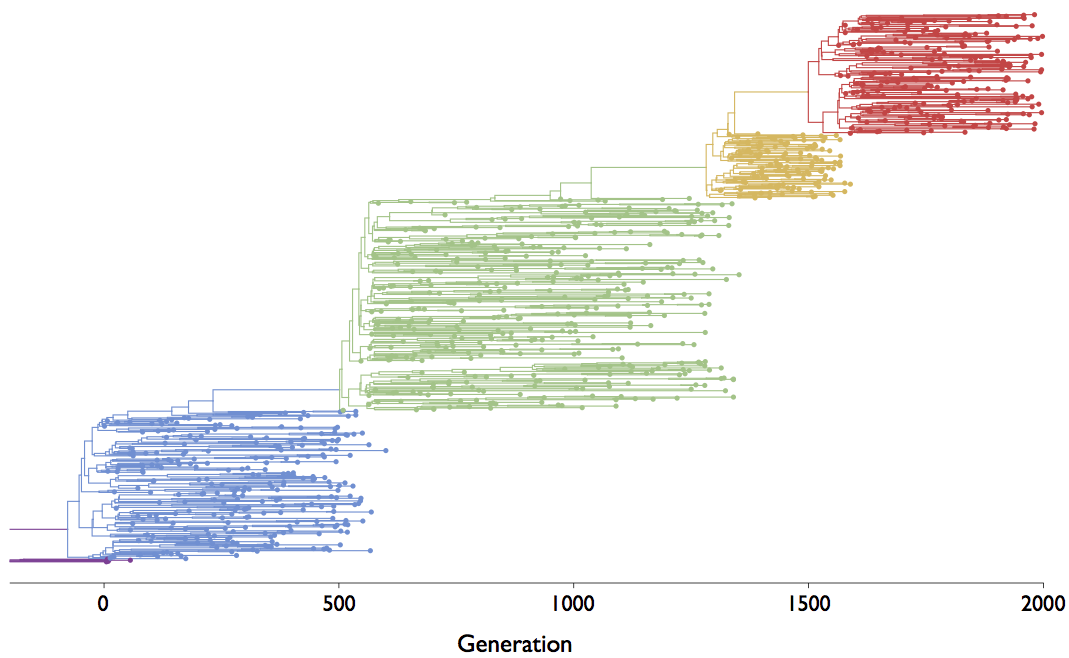## The coalescent

### Epidemic process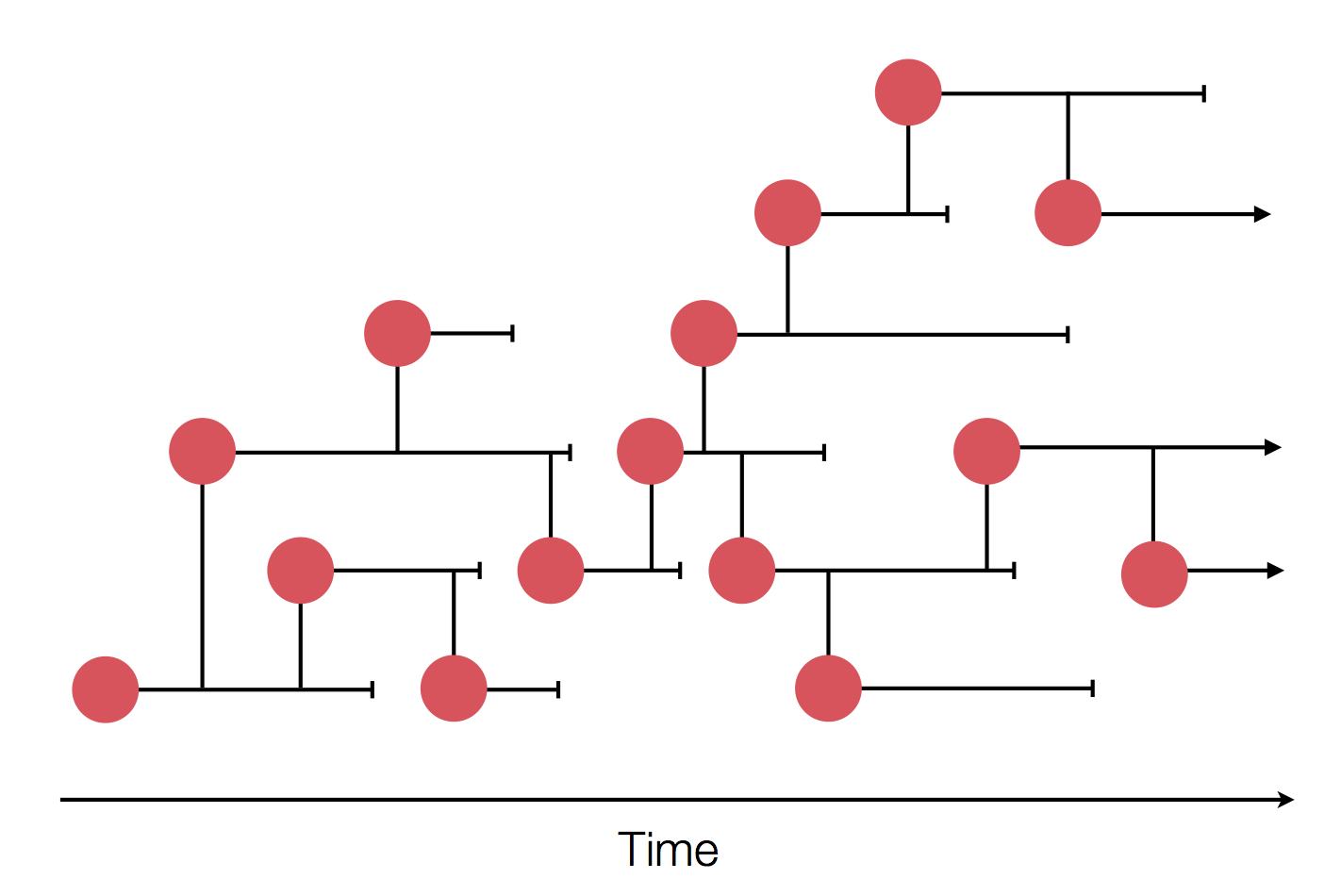### Sample some individuals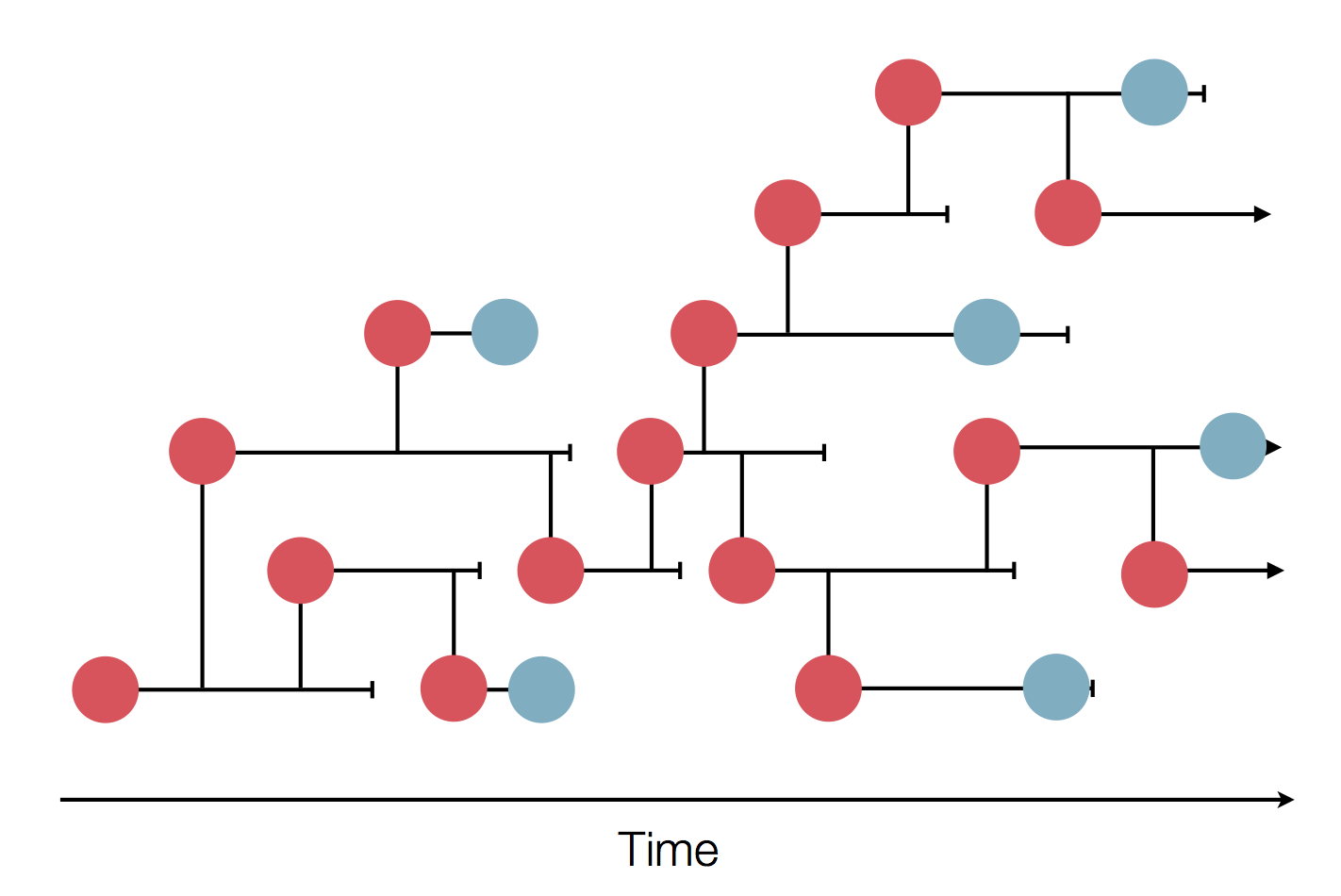### Sequence and determine phylogeny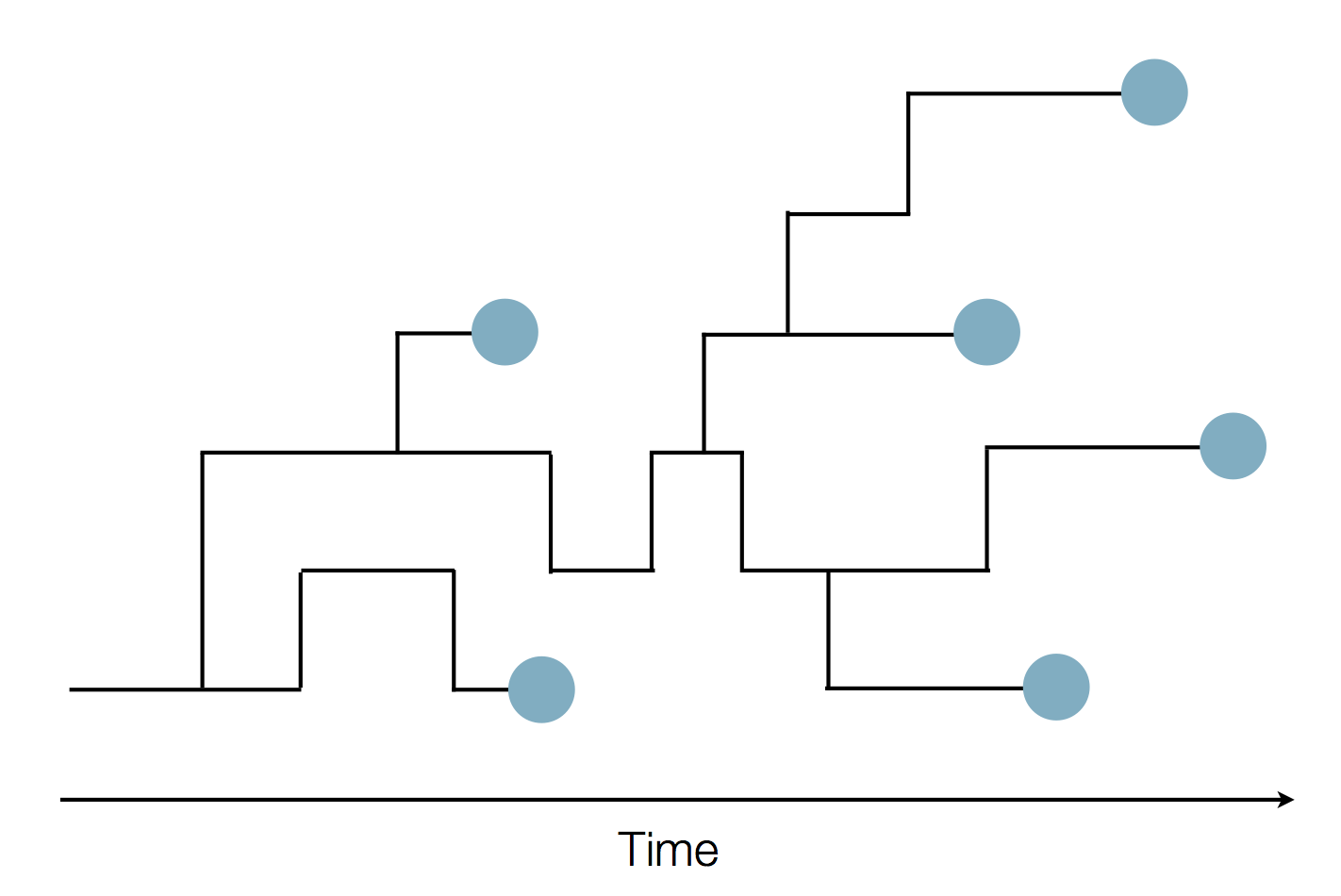### Sequence and determine phylogeny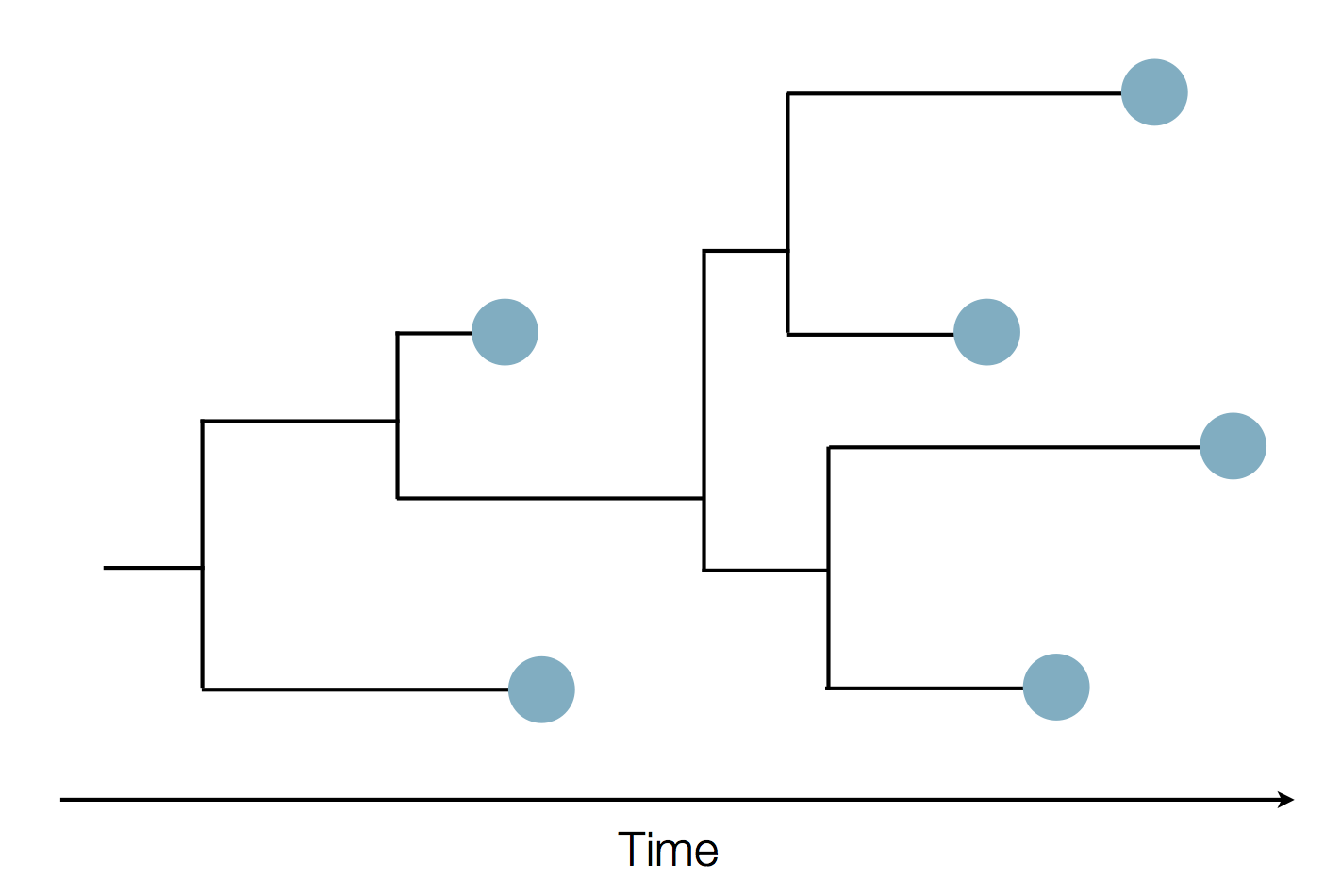### Wright-Fisher process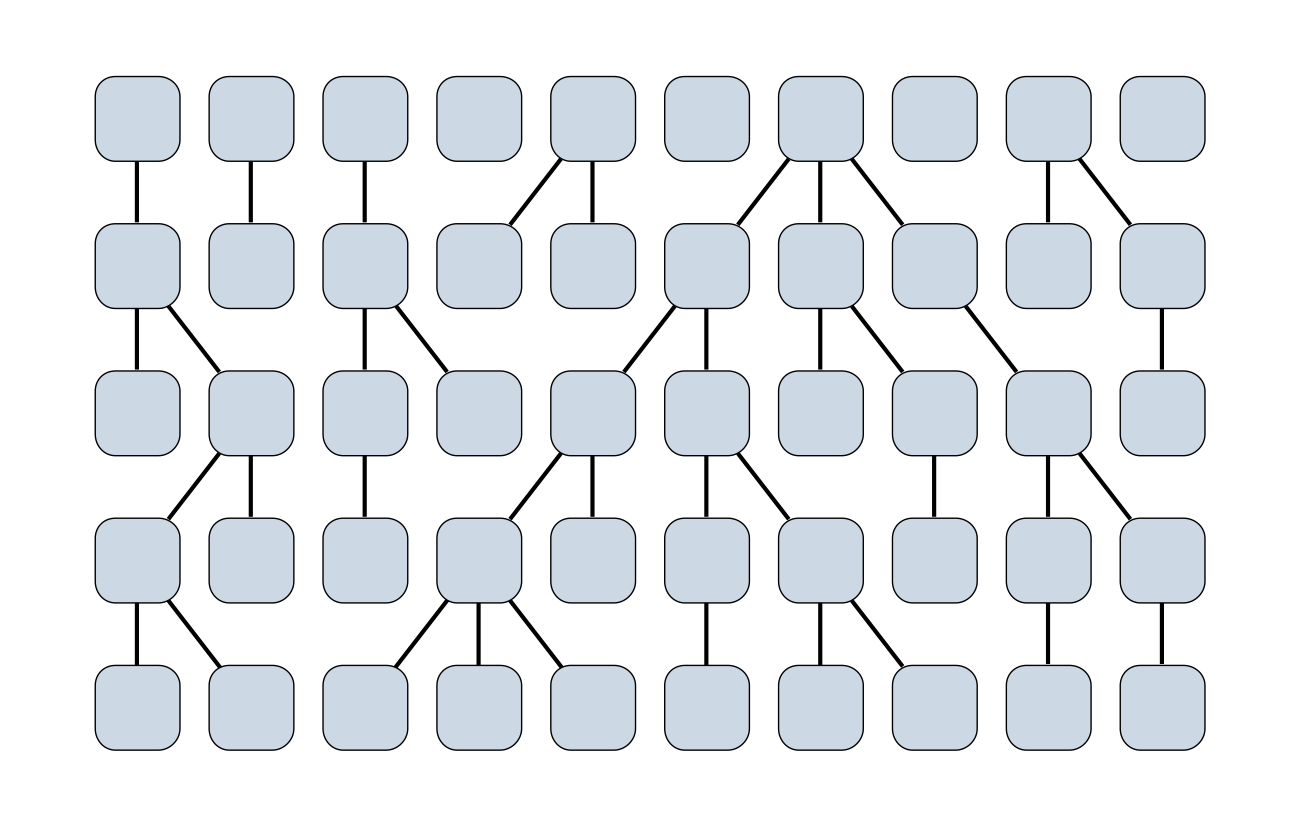### Sample some individuals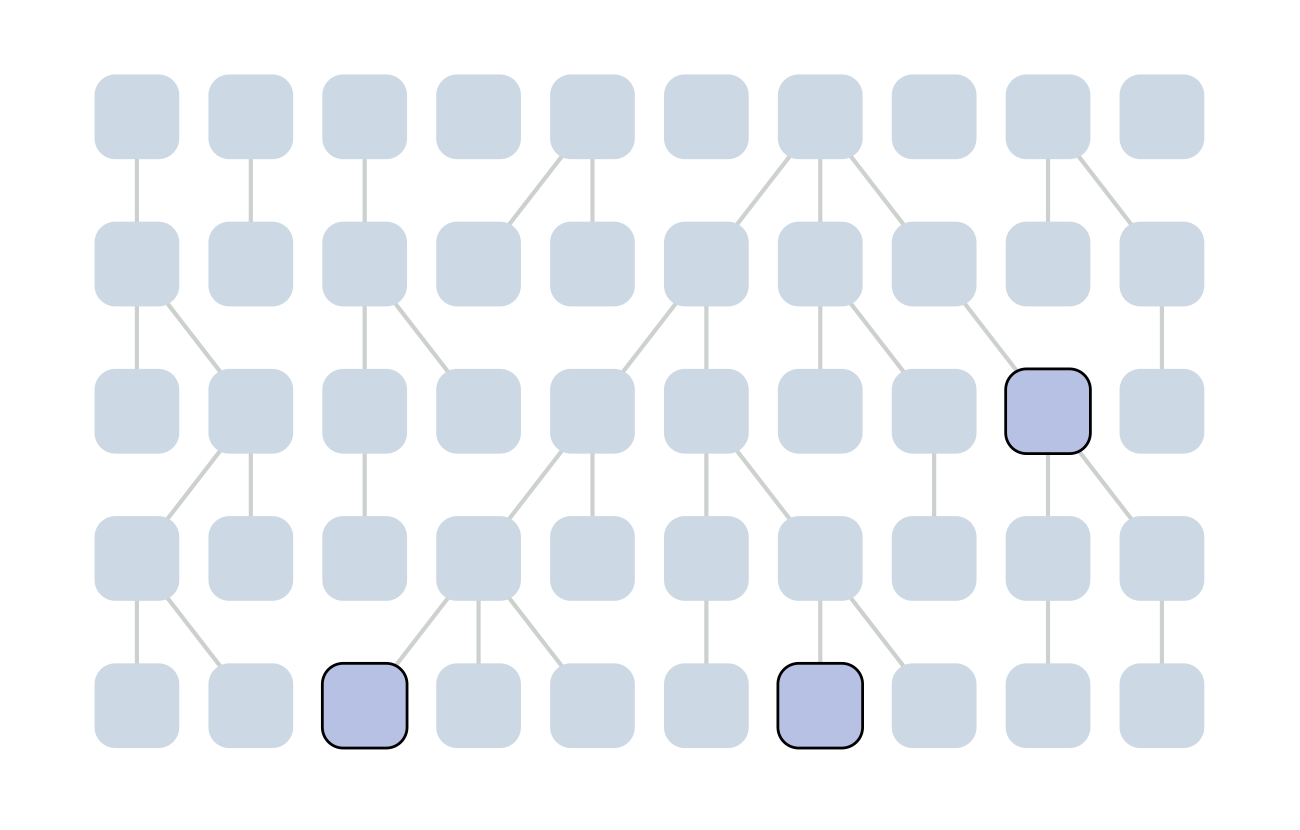### Each generation coalescence may occur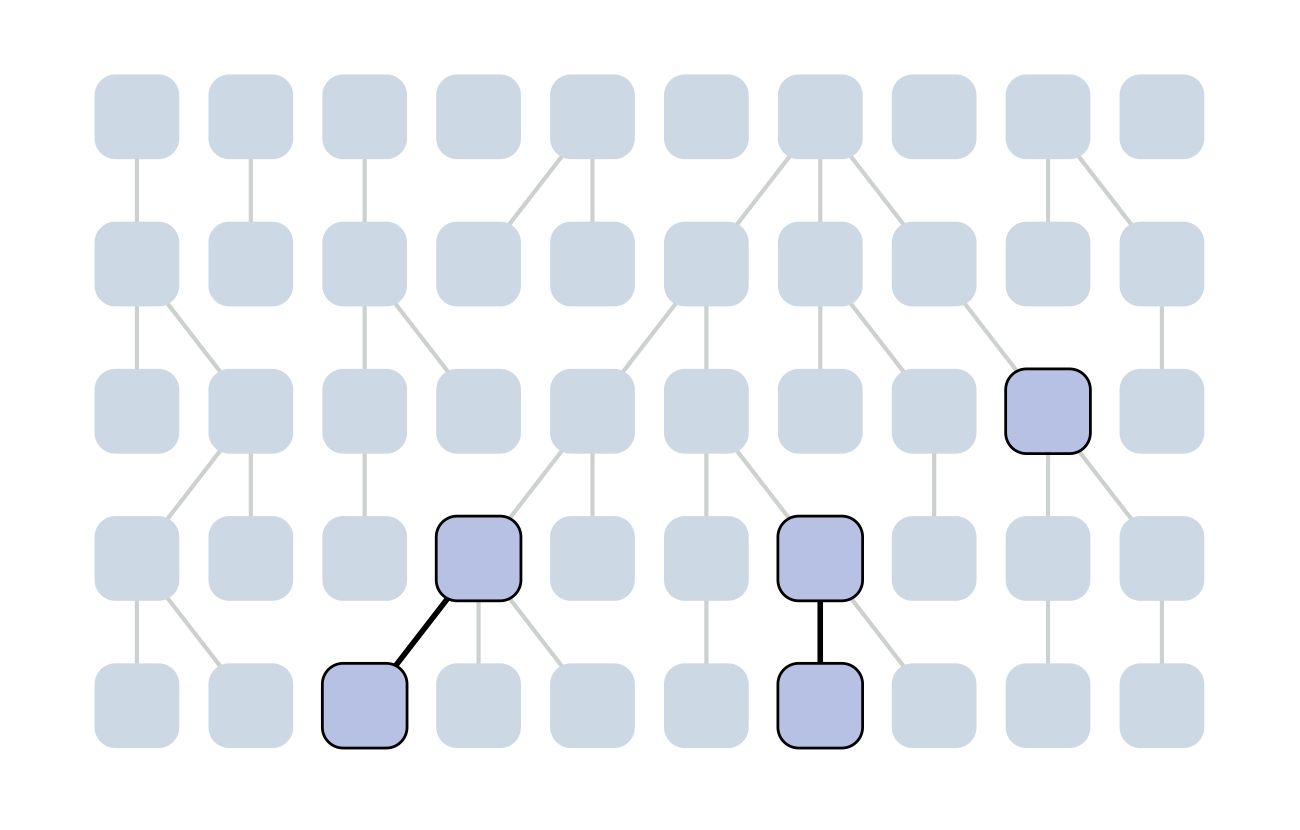### Each generation coalescence may occur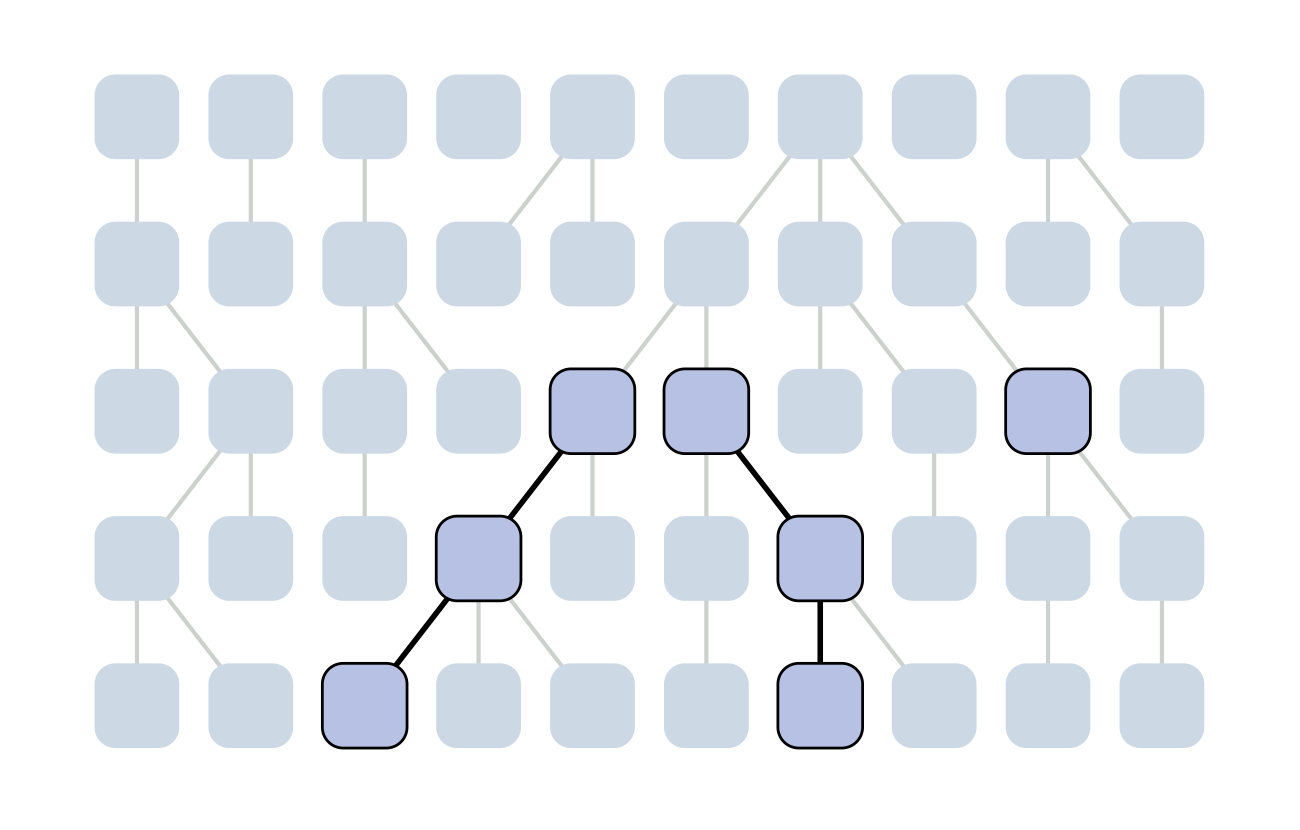### Each generation coalescence may occur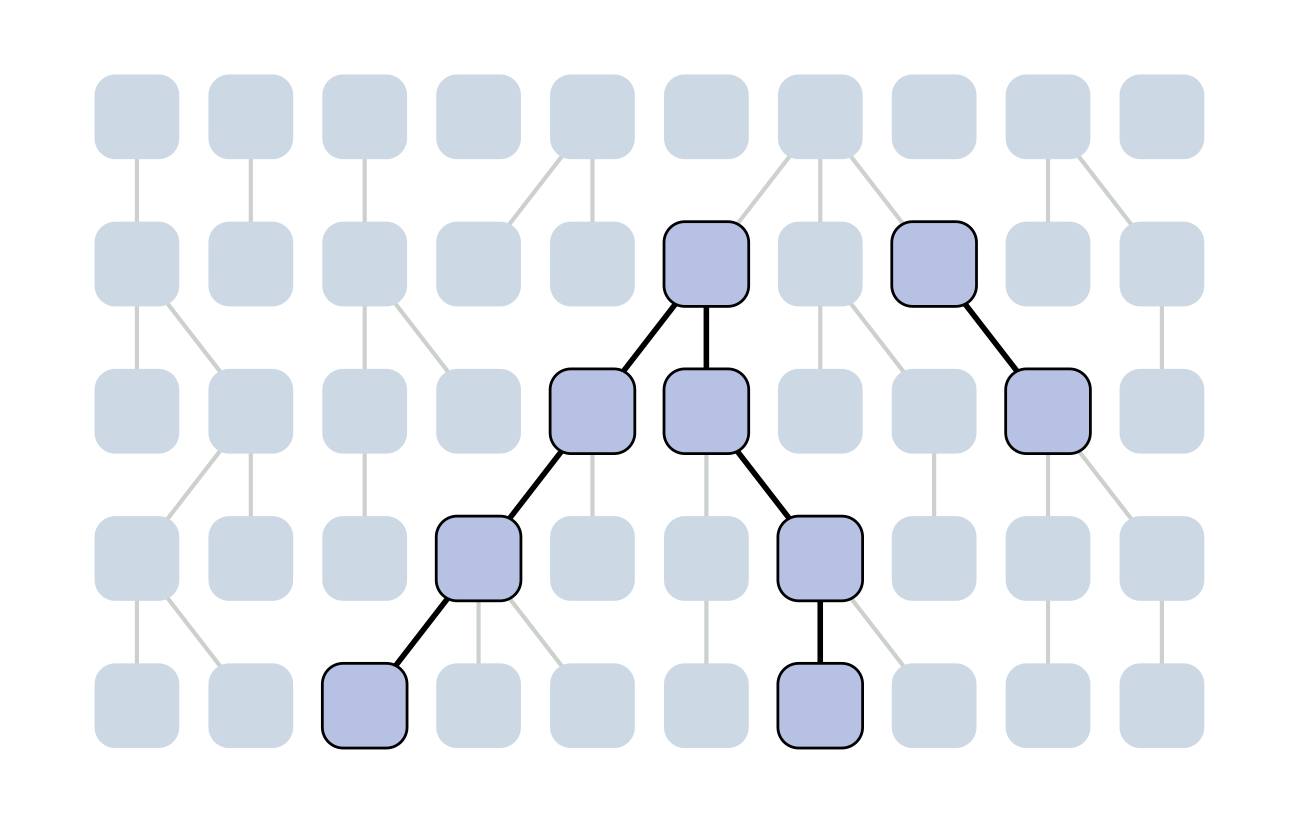### Each generation coalescence may occur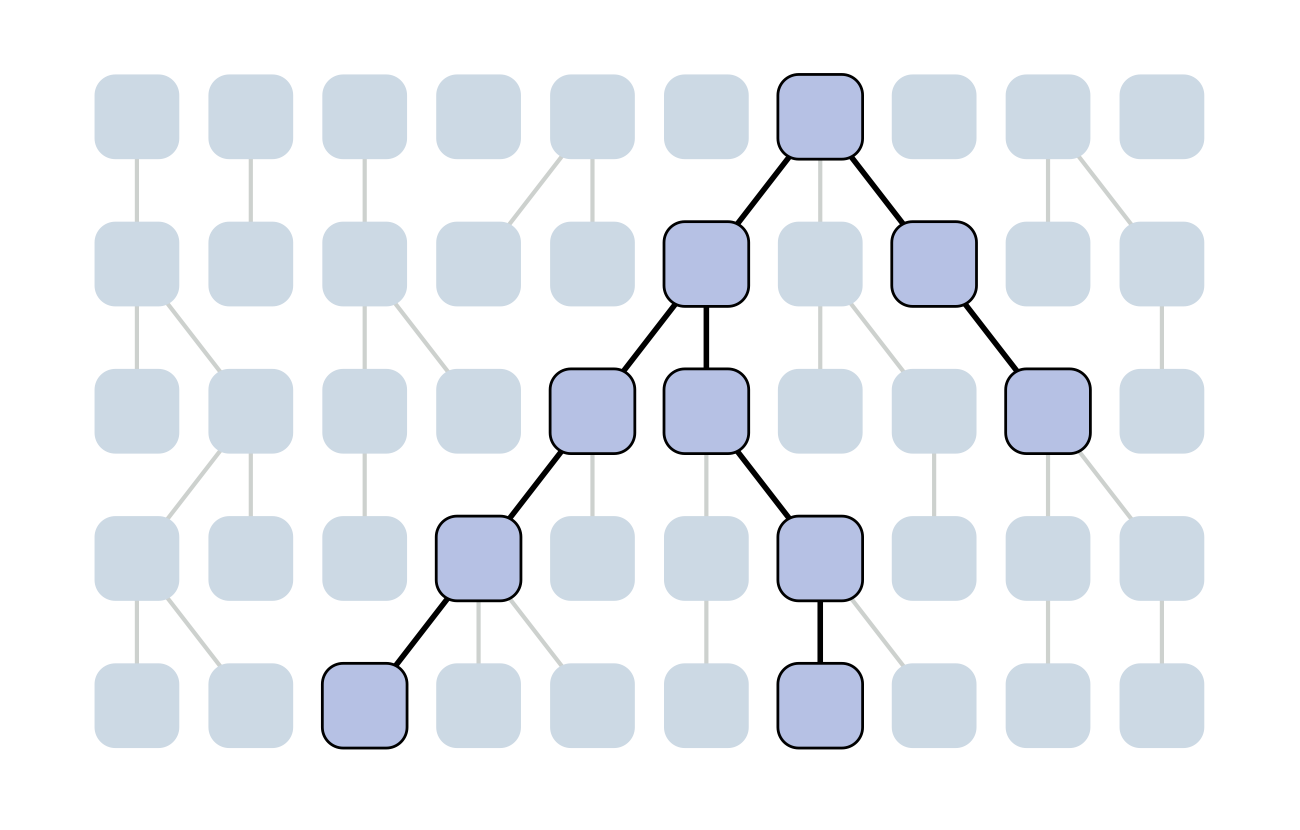### Set of coalescent intervals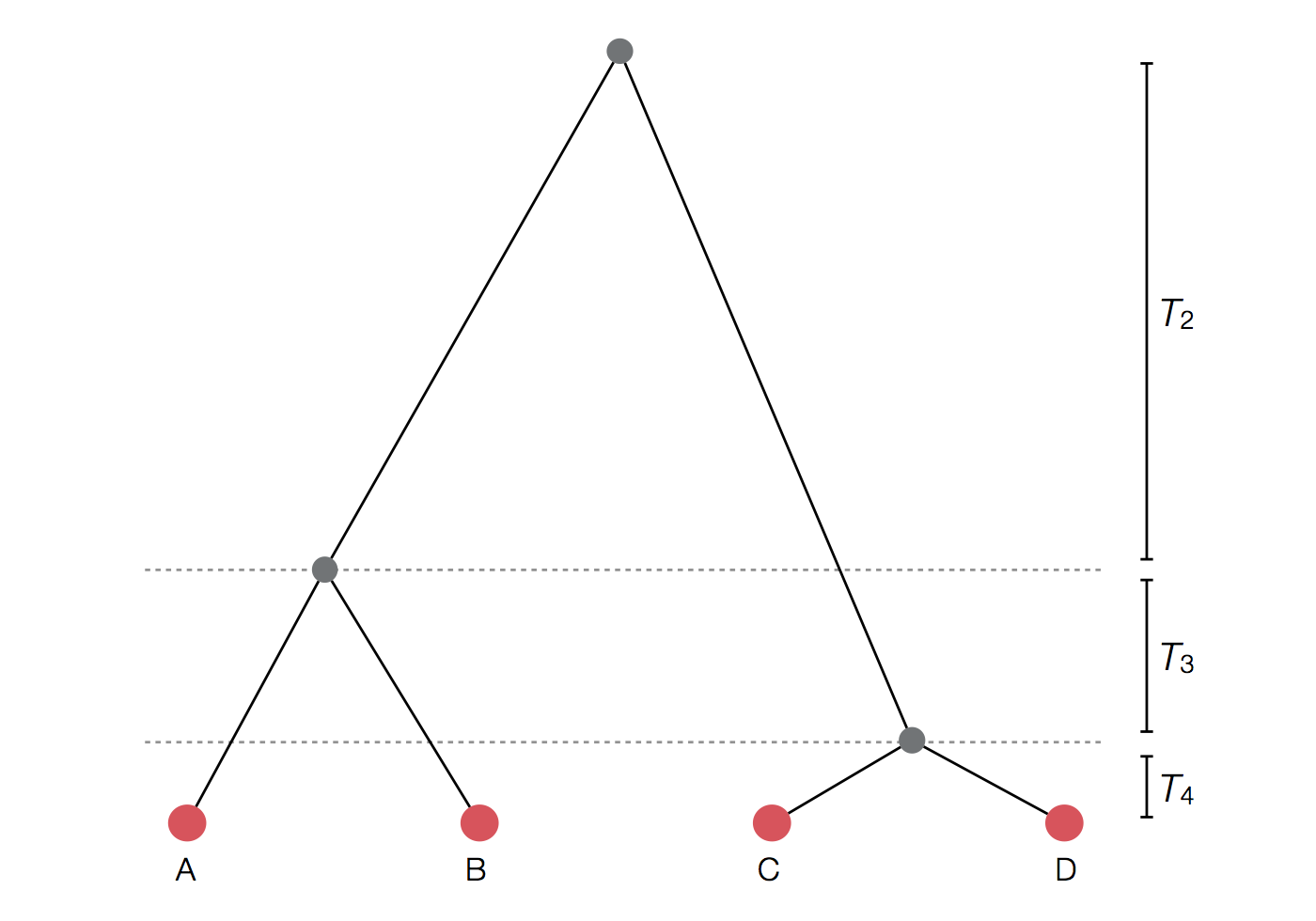### Per-generation probability of coalescence

$$\mathrm{Pr}(\mathrm{coal} \, | \, i=2) = \frac{1}{N}$$

Probability of first lineage picking an arbitrary parent is 1, while the probability of the 2nd lineage picking the same parent is $\frac{1}{N}$.

Probability of coalescence scales inversely with population size.

### Per-generation probability of coalescence

$$\mathrm{Pr}(\mathrm{coal}) = \binom{i}{2} \frac{1}{N} = \frac{i(i-1)}{2N}$$

There are $\binom{i}{2}$ ways pairs of lineages can pick the same parent.

Probability of coalescence scales quadratically with lineage count.

### Expected waiting time to coalescence

$$\mathrm{E}[T_i] = \frac{2N}{i(i-1)}$$

This is a geometric distribution. If each generation there is a $\frac{1}{x}$ probability of an event occurring, we expect to wait $x$ generations for the event to occur.

### Continuous time limit

With per-generation probability of an event $\frac{1}{x}$ small, but many generations, then the discrete time geometric distribution approximates to a continuous time exponential distribution. Thus, we assume $T_i$ to be exponentially distributed with mean

$$\mathrm{E}[T_i] = \frac{2N}{i(i-1)}.$$

### Set of coalescent intervals with waiting times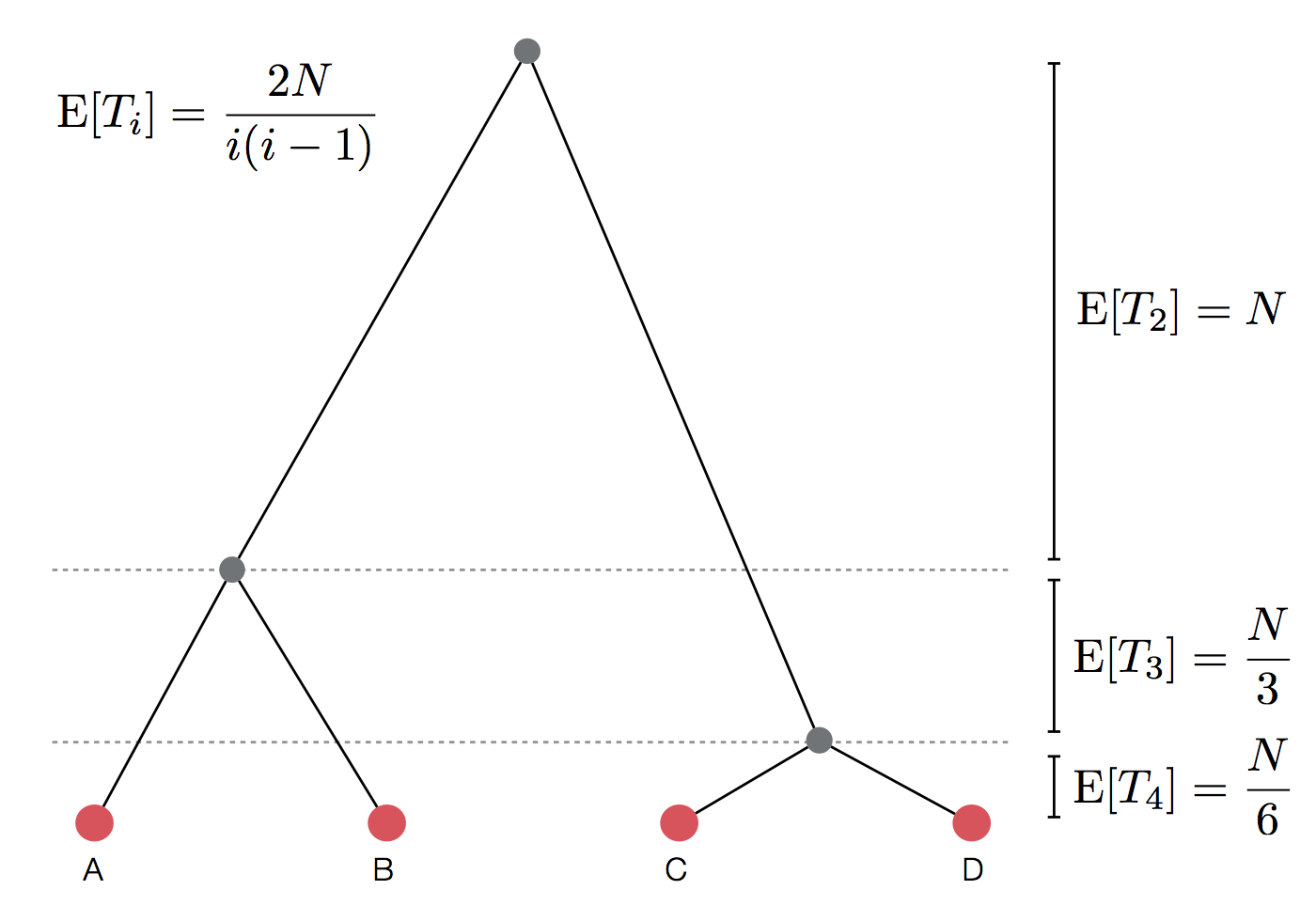### Gives coalescent trees their distinctive shape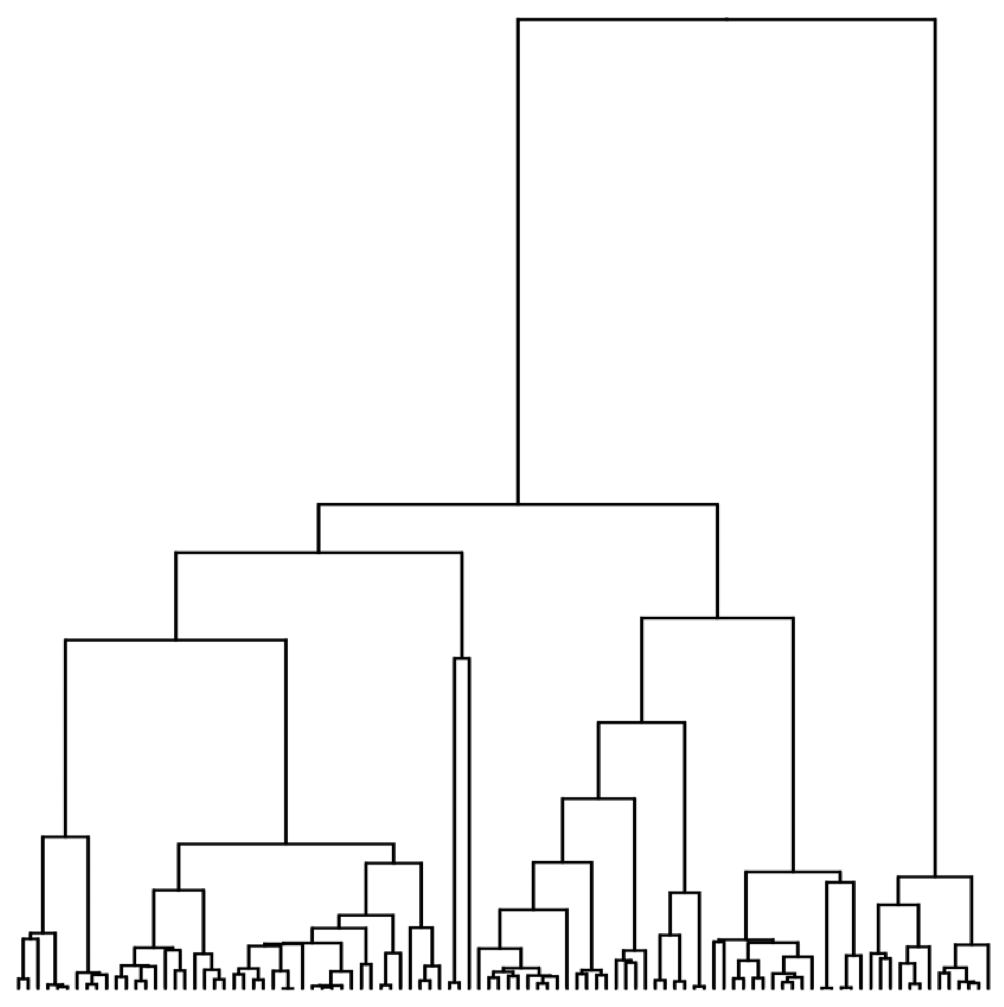### Rate of coalescence scales with population size N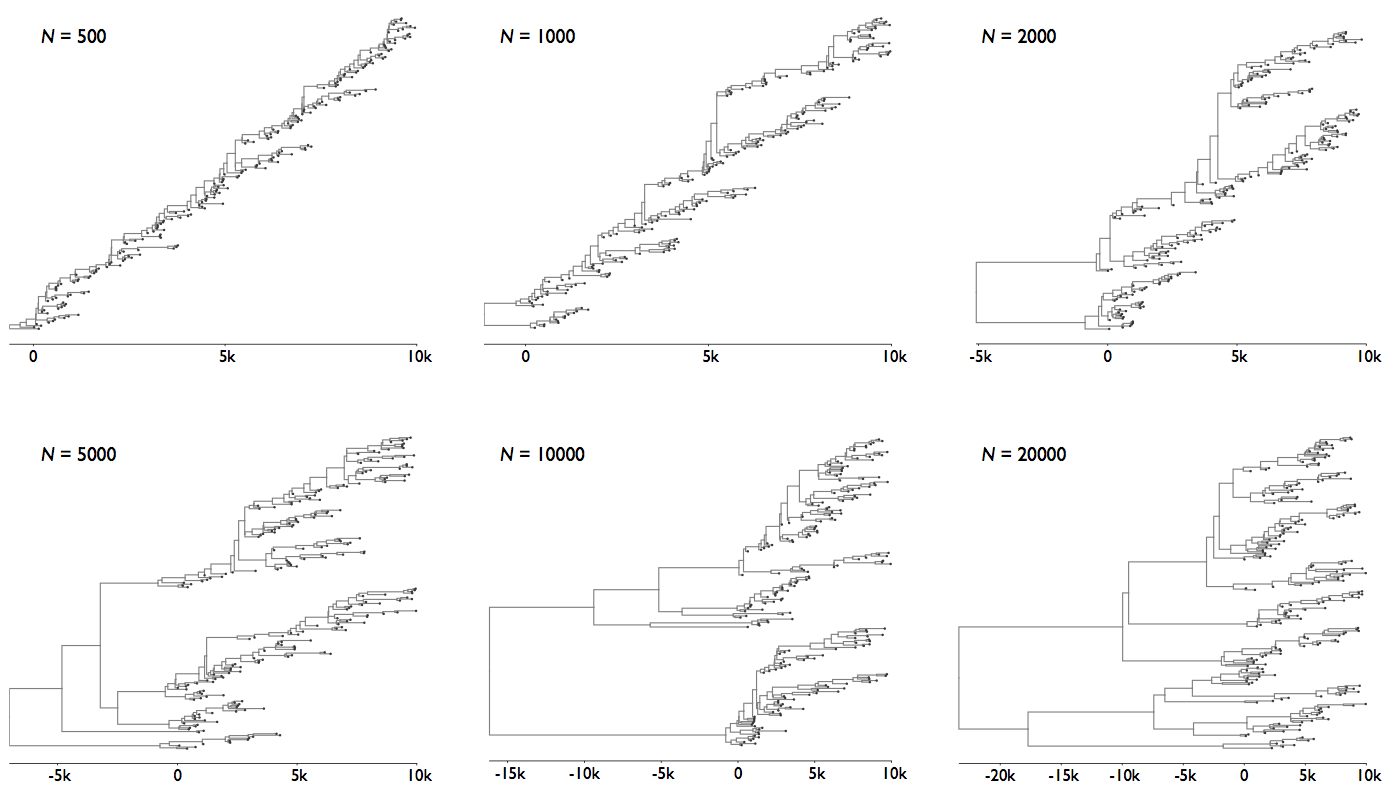## Visualization of the coalescent process

### Pairwise genetic diversity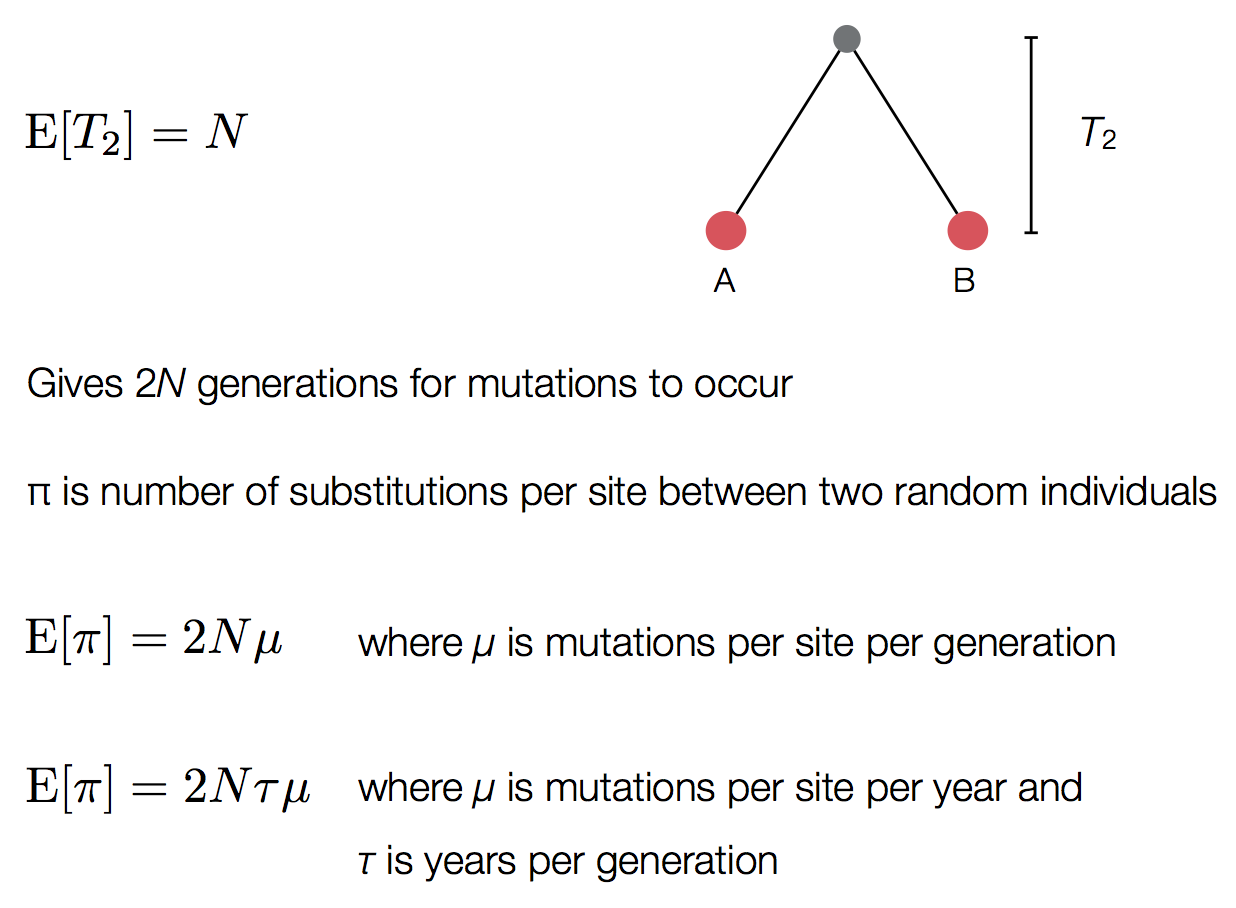### Time to the most recent common ancestor (TMRCA)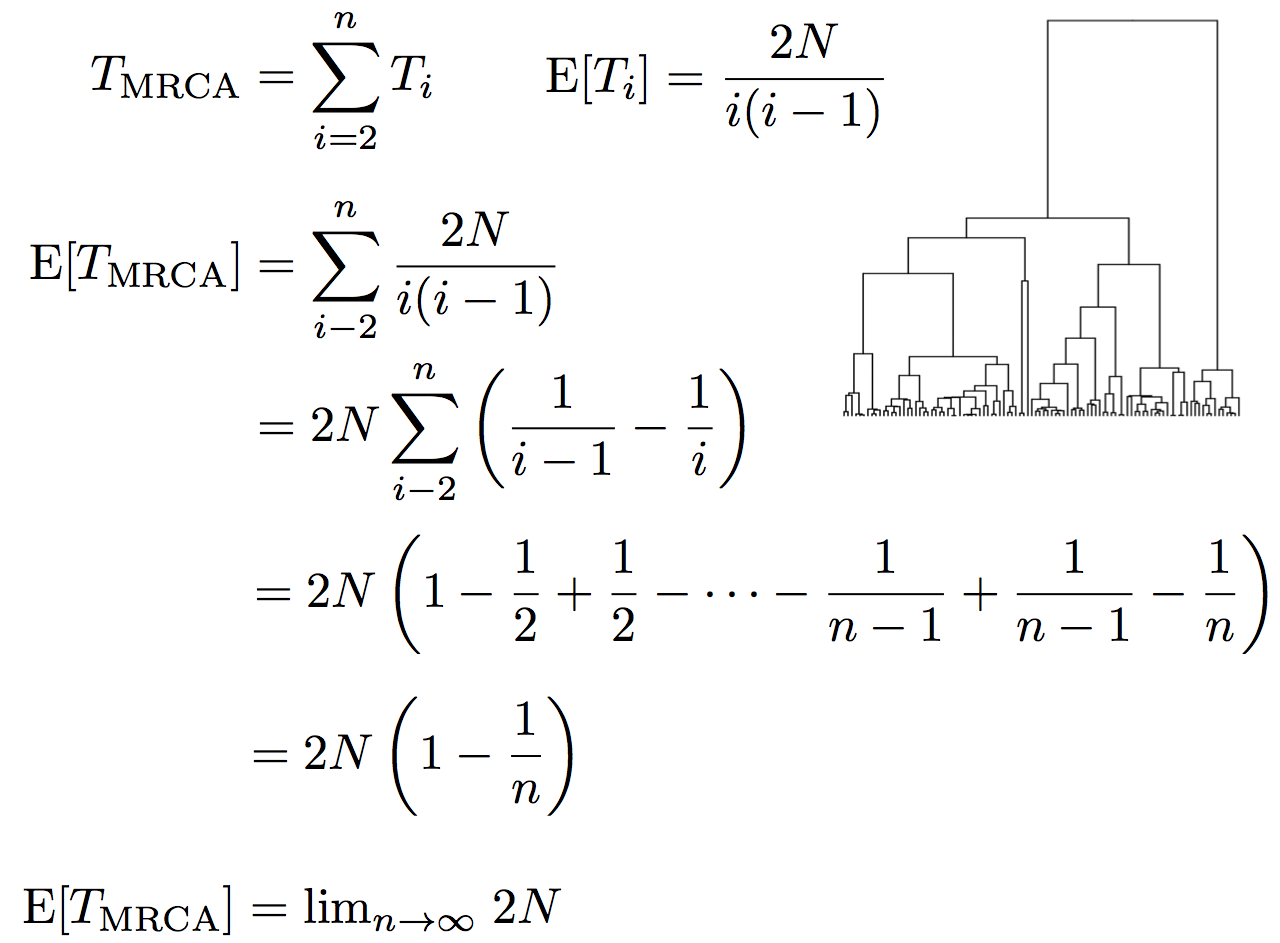## Coalescence patterns can estimate population growth/decline

### Changing population size alters coalescent rate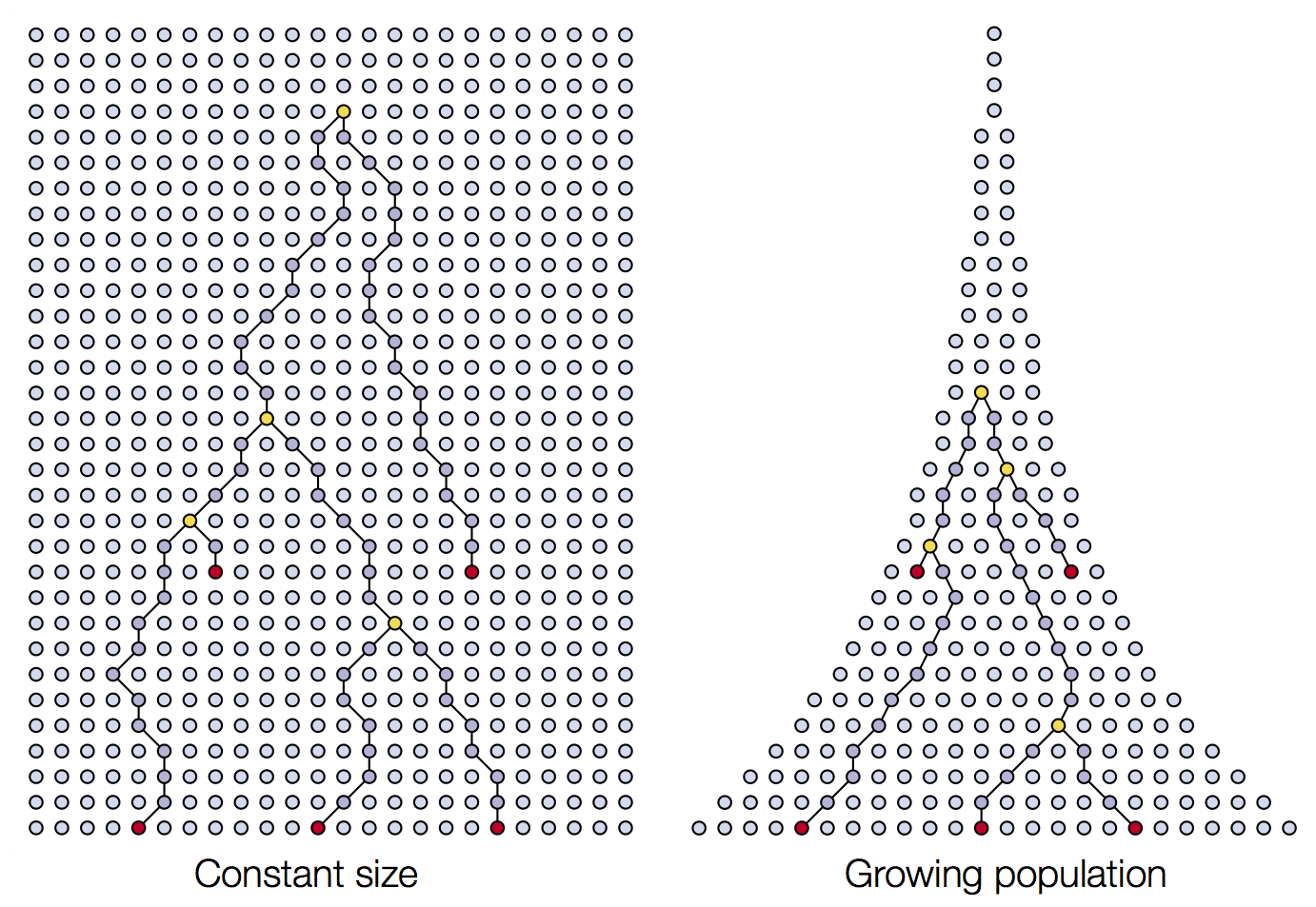### Changing population size alters coalescent rate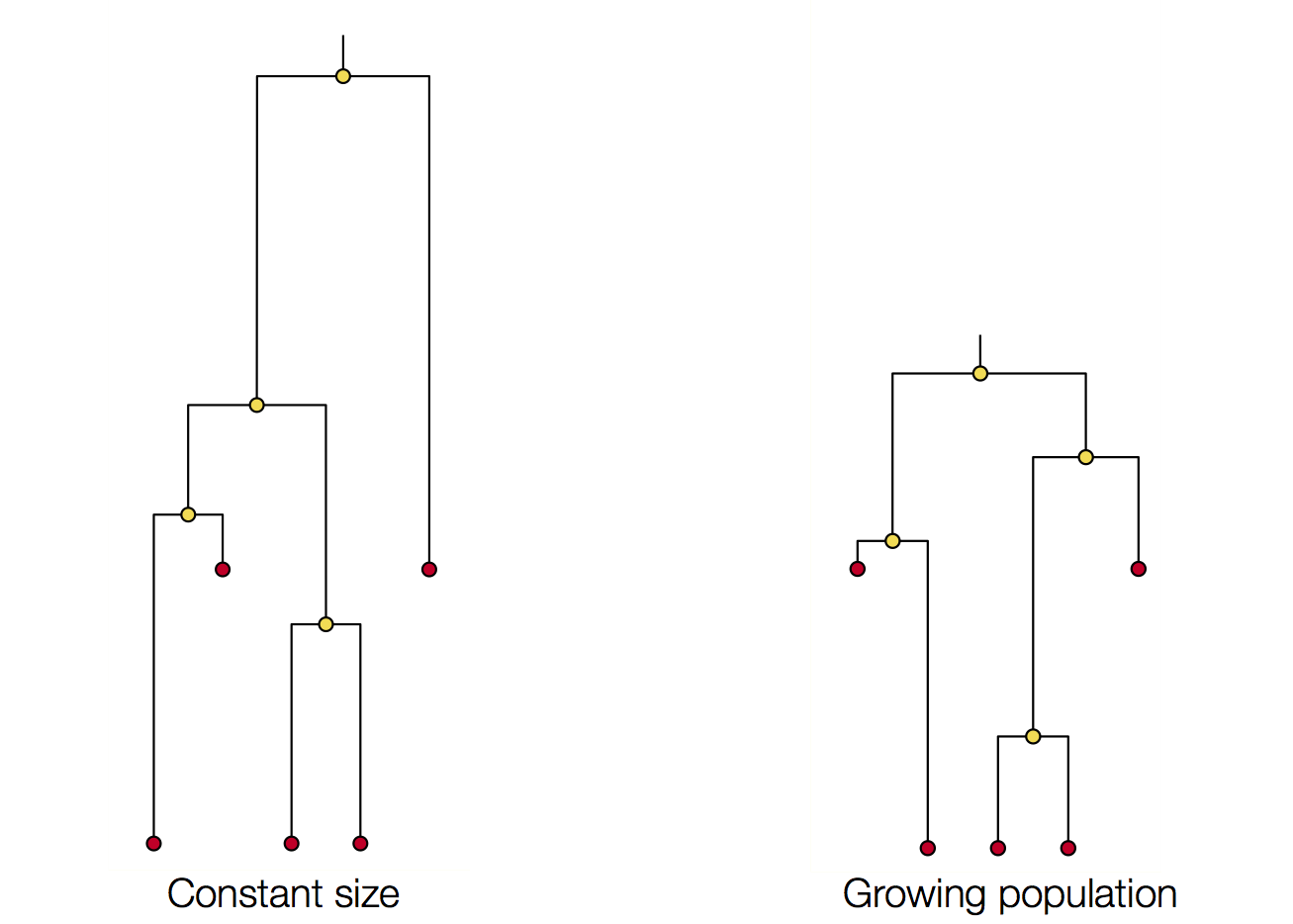### 'Skyline' is flexible demographic model that estimates windows of coalescent rate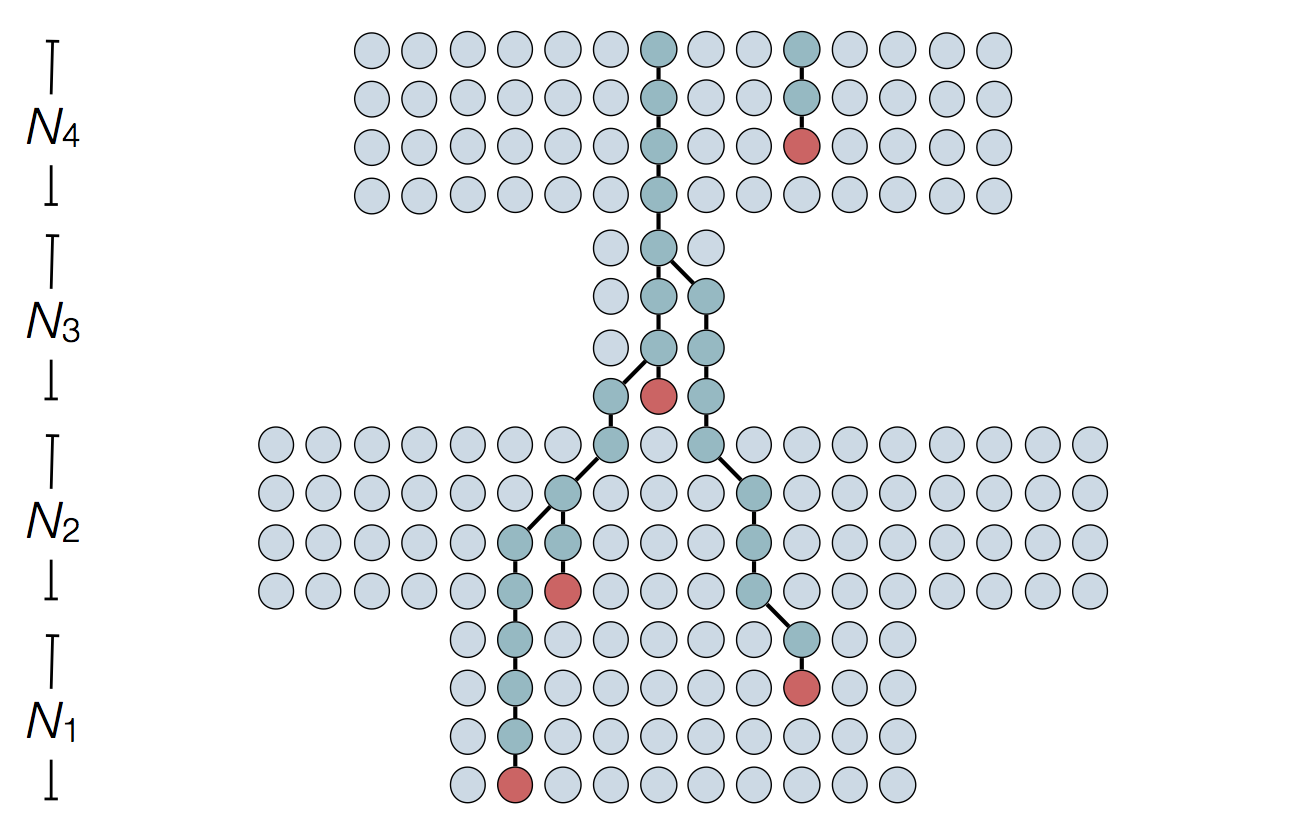### Skyline model shows population growth in HCV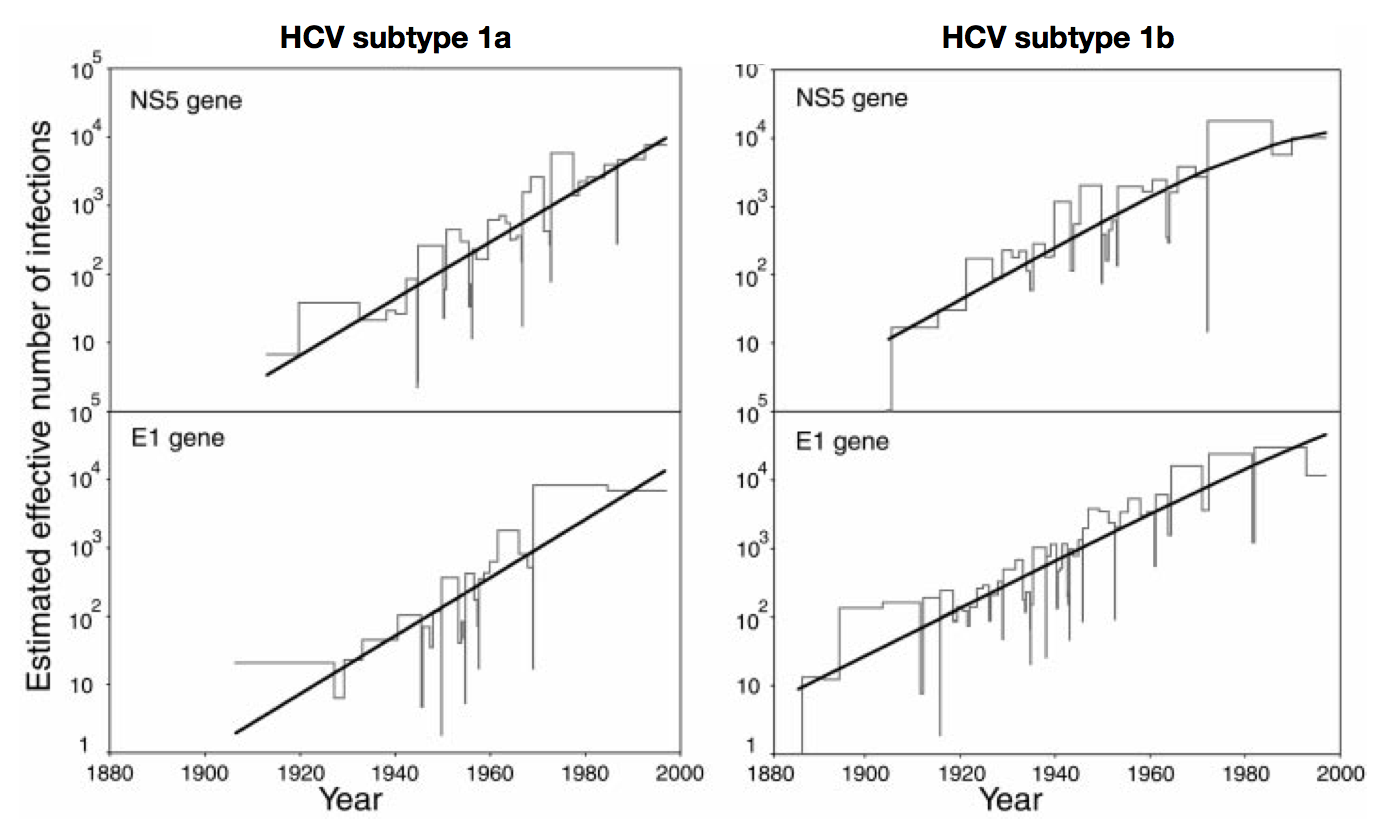### Skyline model shows seasonality in flu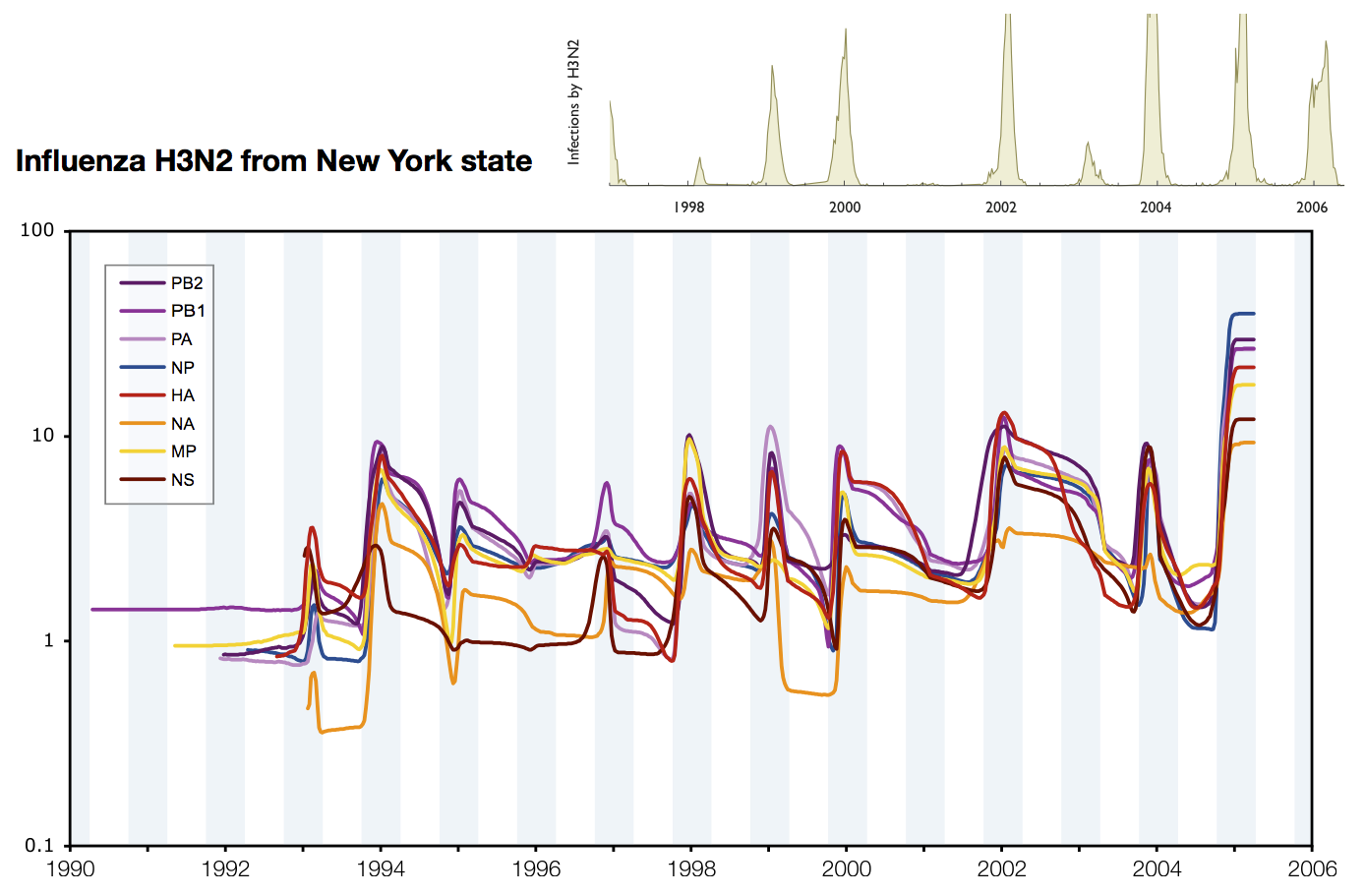### Interpreting rate of coalescence

These approaches directly estimate the pairwise rate of coalescence $\lambda$, which is measured in terms of events per year. Thus, the timescale of coalescence $\frac{1}{\lambda}$ is measured as the expected waiting time in years for two lineages to find a common ancestor.

### Interpreting rate of coalescence

The timescale of coalescence $\frac{1}{\lambda}$ is equal to $N_e\tau$, where $N_e$ is measured in generations and $\tau$ is measured in years per generation. $\tau$ acts to rescale time from generations to years.

### Example tree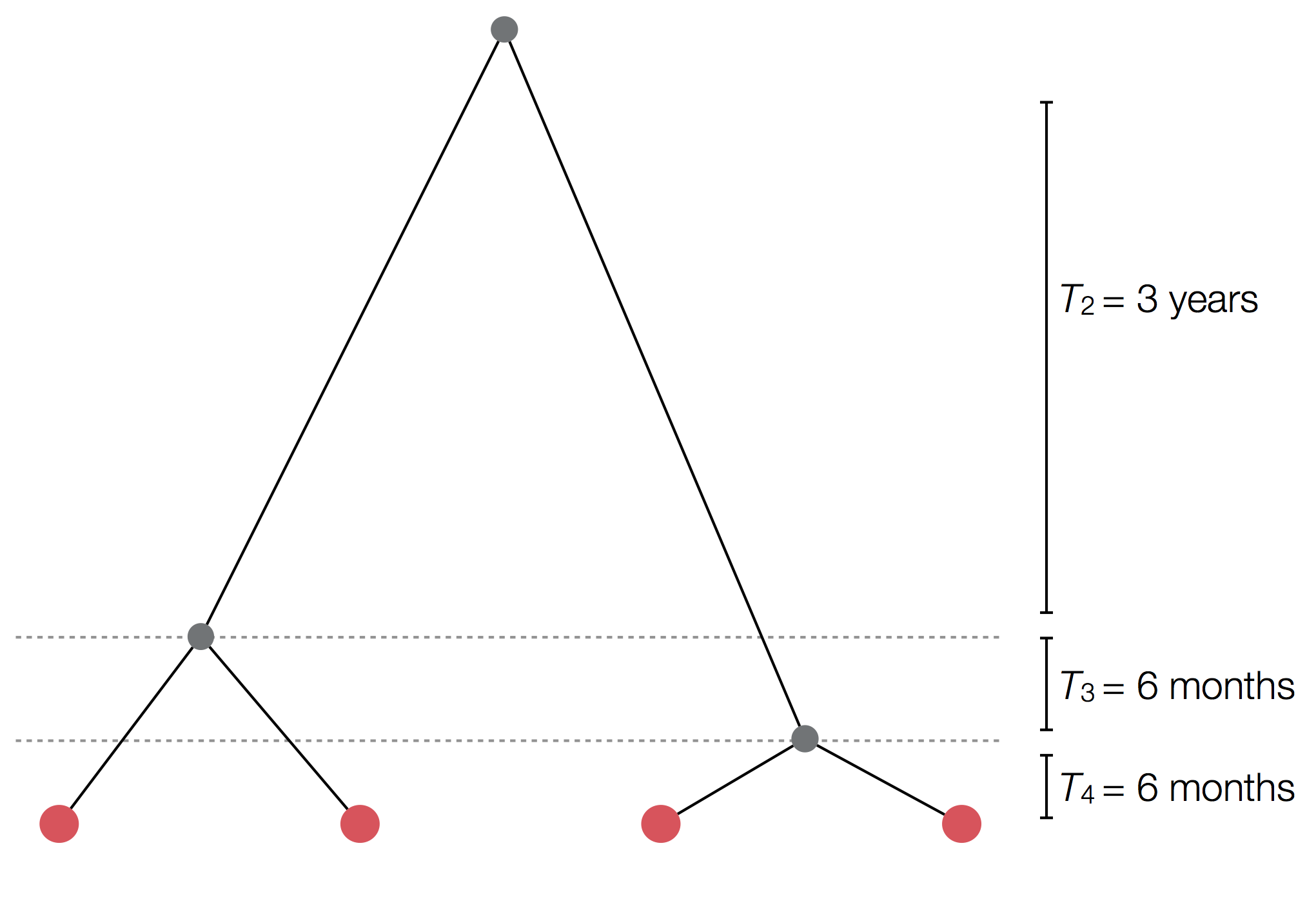### Example skyline plot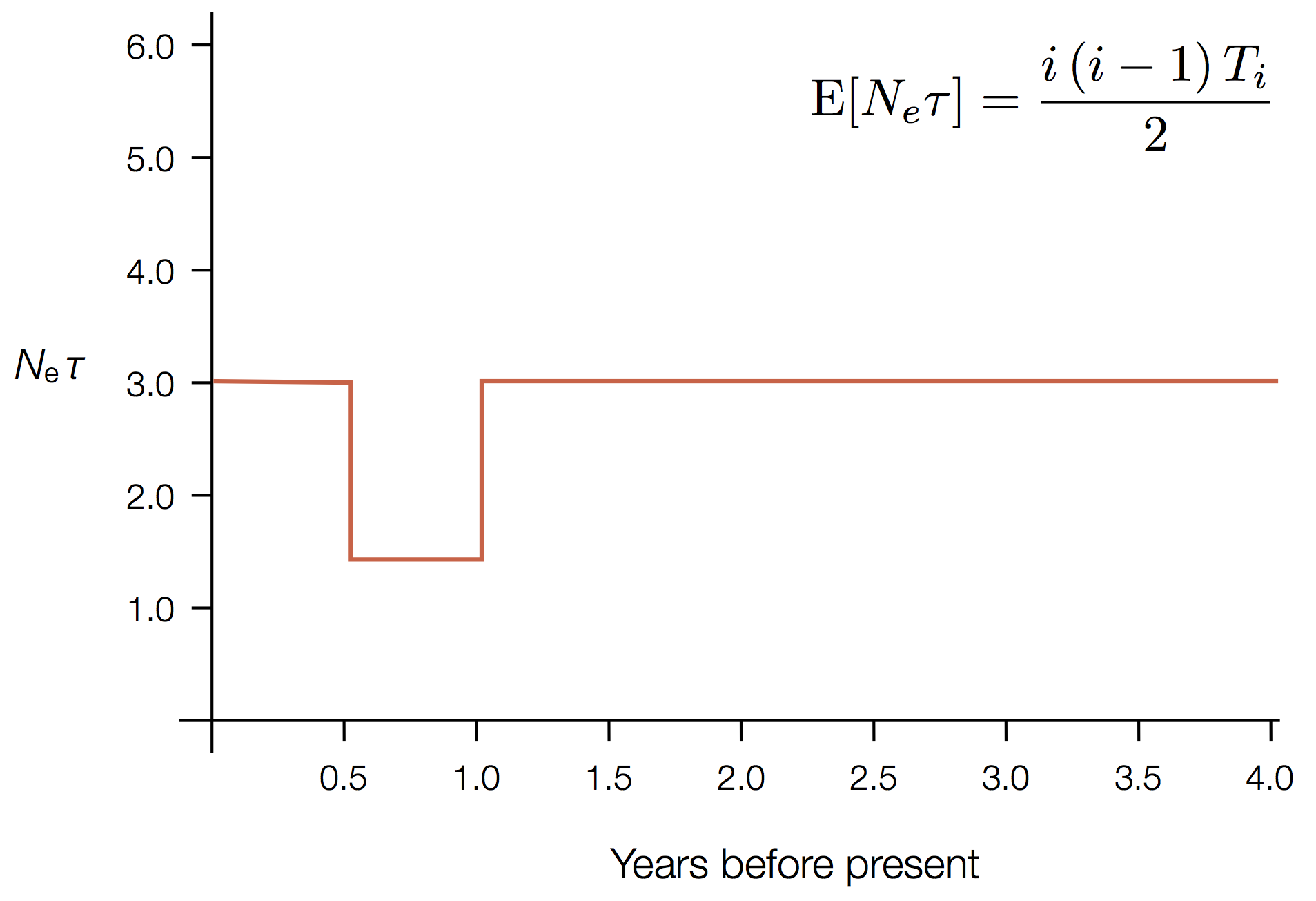### Exercise: plot skyline from tree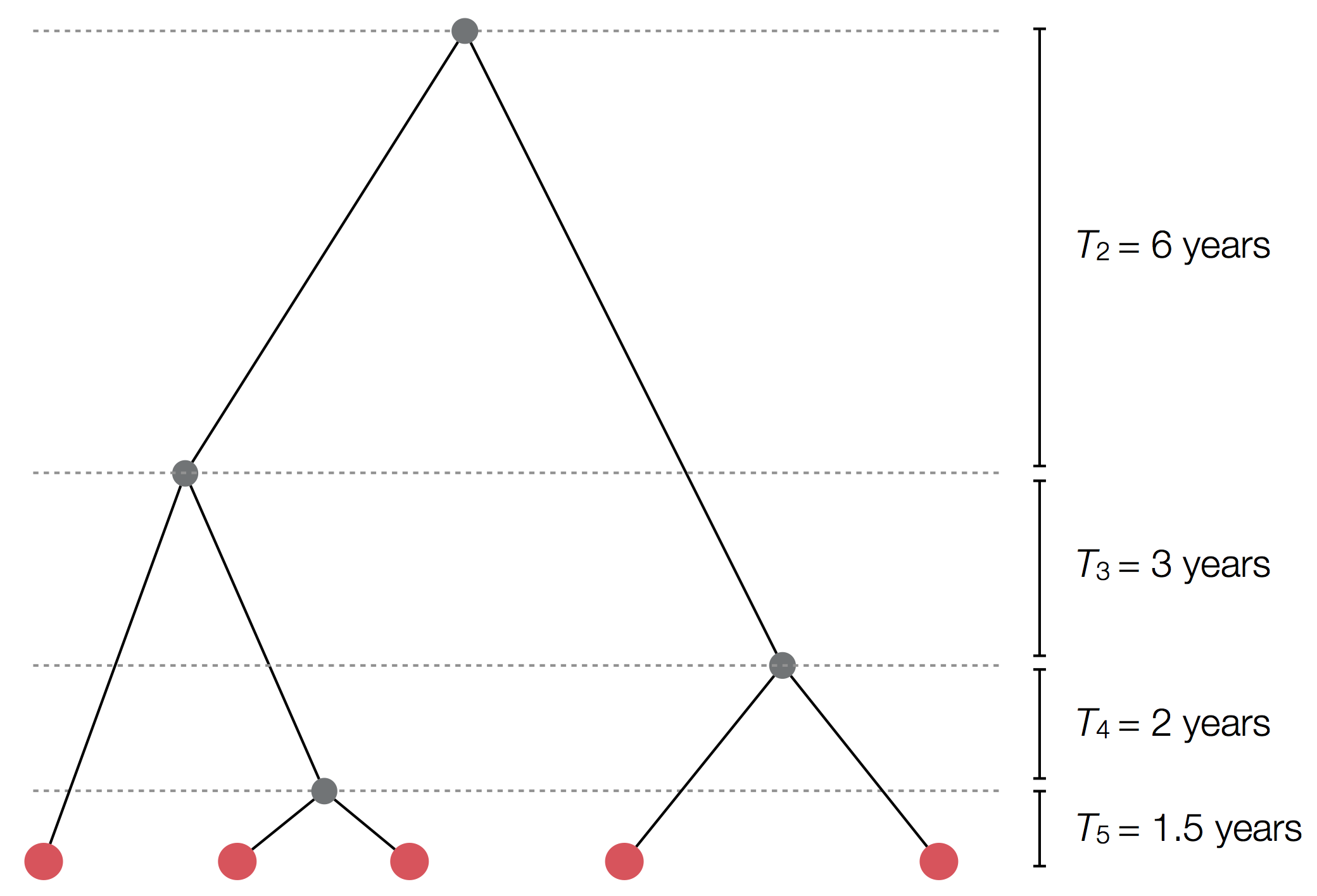## Selection distorts coalescence patterns

### Neutral population dynamics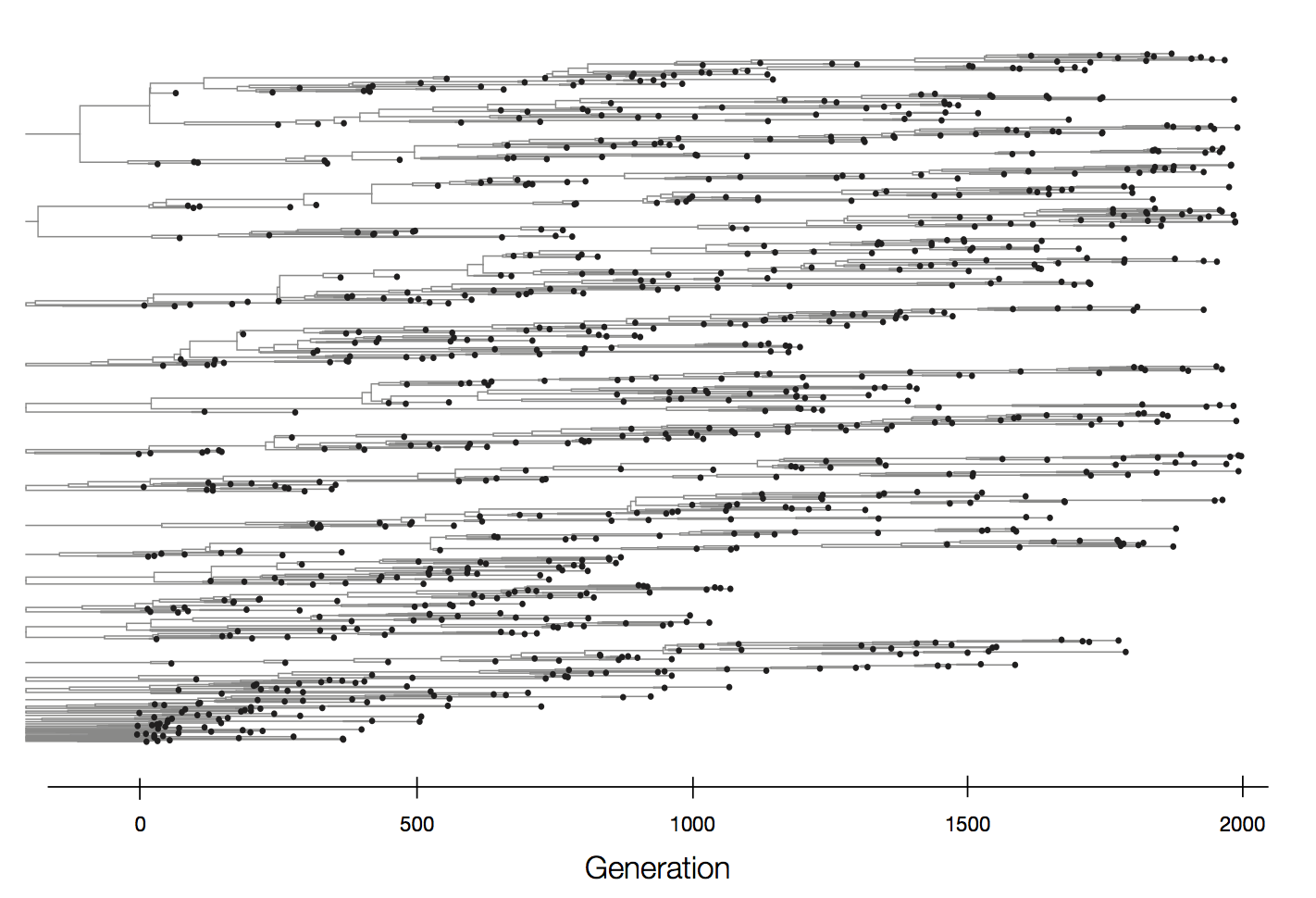### Purifying selection reduces genetic diversity and leads to population stasis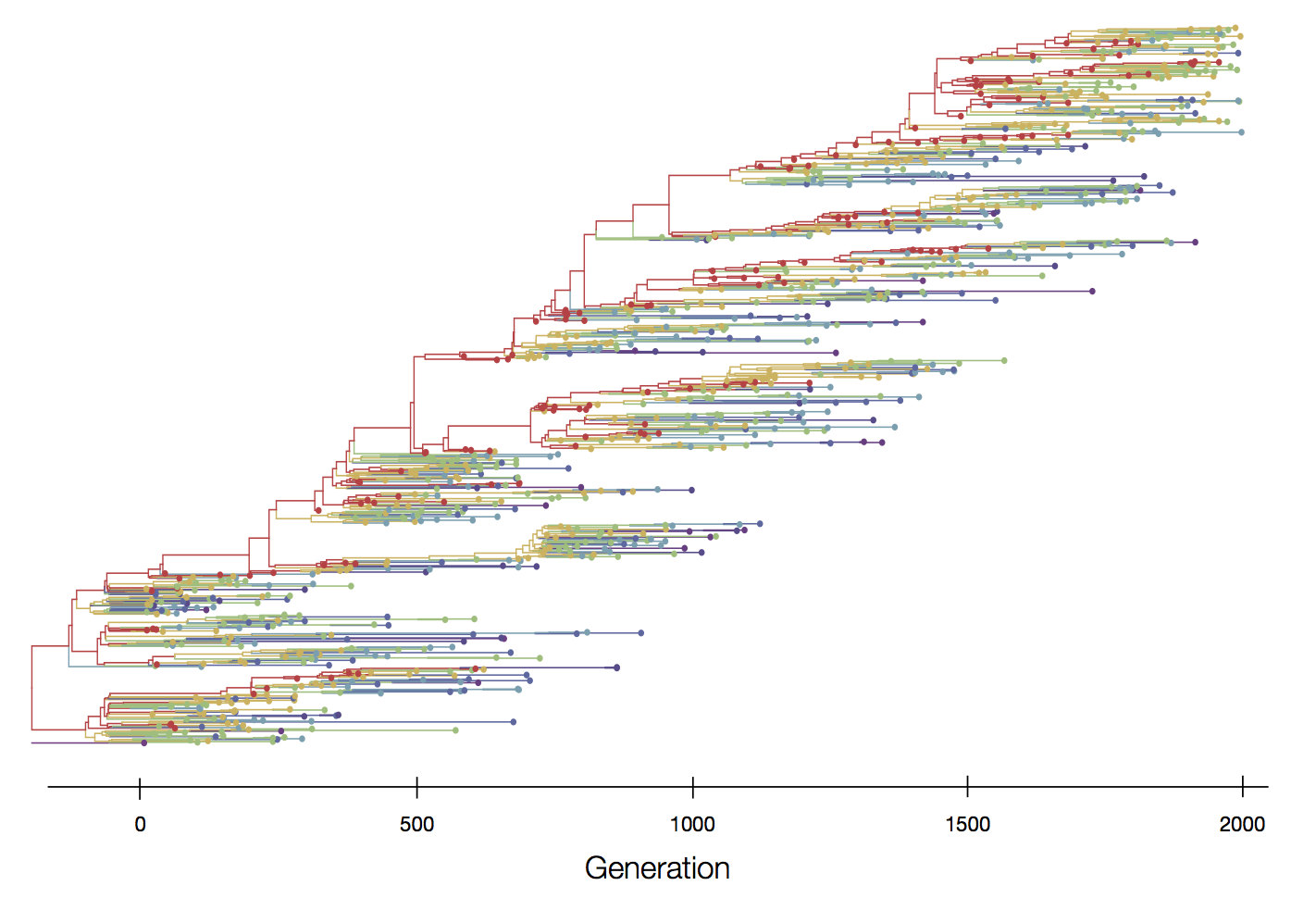### Positive selection reduces genetic diversity leads to population turnover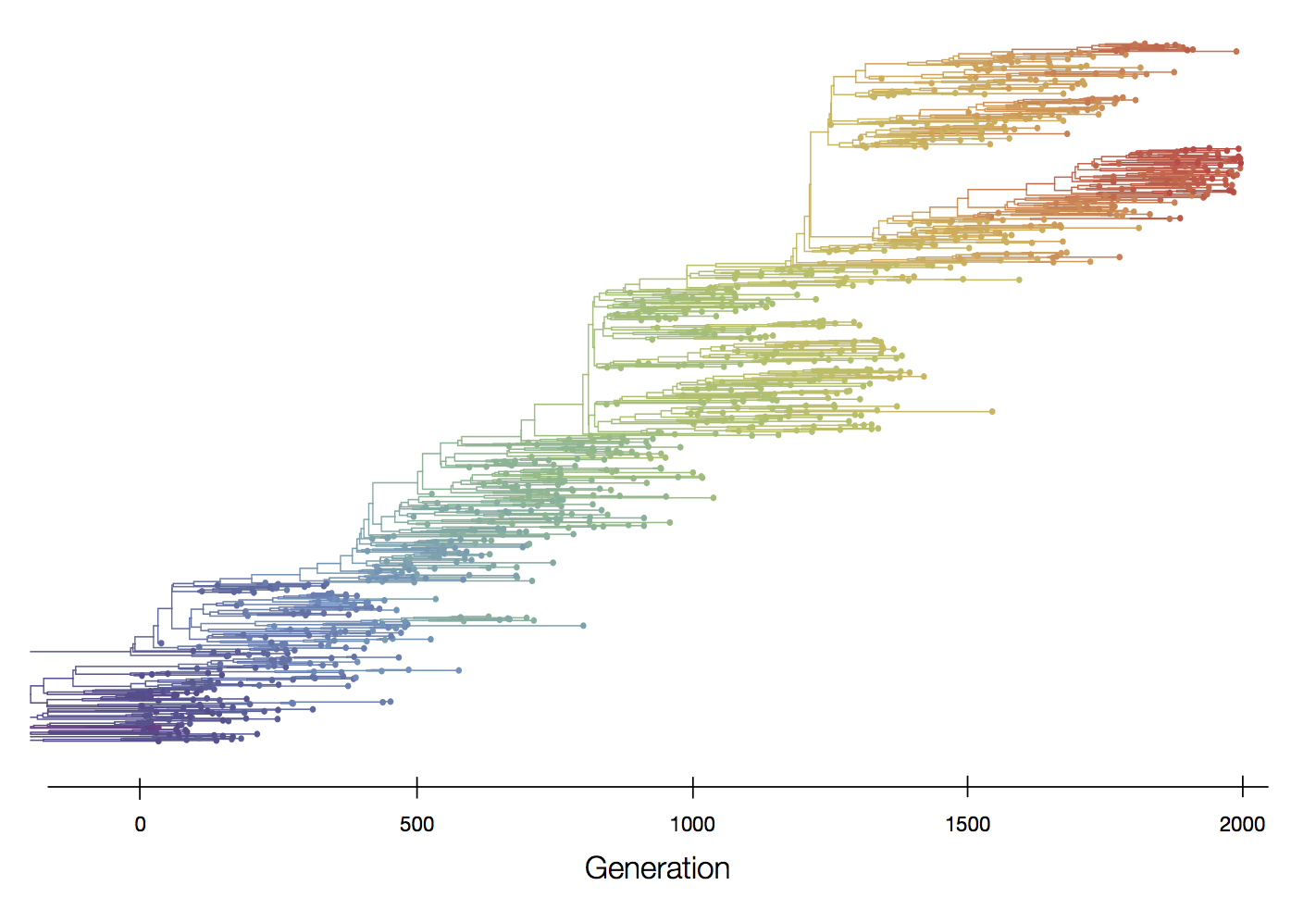### Episodic positive selection shows selective sweeps